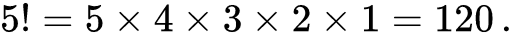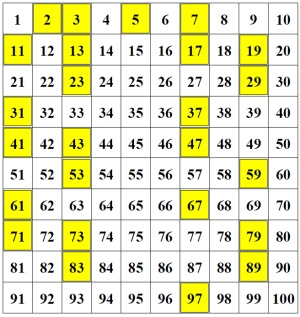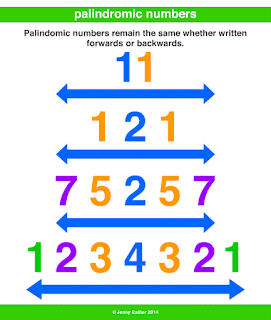## Posts

### Factorial NumberFACTORIAL NUMBER
The factorial of a positive integer n, denoted by n!, is the product of all positive integers less than or equal to n. For example, {\displaystyle 5!=5\times 4\times 3\times 2\times 1=120\,.}The value of 0! is 1, according to the convention for an empty product Mathematically, the formula for the factorial is as follows. If n is an integer greater than or equal to 1, then n ! = n ( n - 1)( n - 2)( n - 3) ... (3)(2)(1) If p = 0, then p ! = 1 by convention.
We can easily calculate a factorial from the previous one: As a table: nn!111122 × 1= 2 × 1!= 233 × 2 × 1= 3 × 2!= 644 × 3 × 2 × 1= 4 × 3!= 2455 × 4 × 3 × 2 × 1= 5 × 4!= 1206etcetc
C Program for Factorial of a Number
#include<stdio.h>int main(){int n, i;unsignedlonglong factorial =1; printf("Enter an integer: "); scanf("%d",&n);// show error if the user enters a negative integerif

### Prime NumberPRIME NUMBER A prime number is a whole number greater than 1 whose only factors are 1 and itself. A factor is a whole numbers that can be divided evenly into another number. The first few prime numbers are 2, 3, 5, 7, 11, 13, 17, 19, 23 and 29. Numbers that have more than two factors are called composite numbers. The number 1 is neither prime nor composite.
For every prime number p, there exists a prime number p' such that p' is greater than p.  This mathematical proof, which was demonstrated in ancient times by the Greek mathematician Euclid, validates the concept that there is no "largest" prime number. As the set of natural numbers N = {1, 2, 3, ...} proceeds, however, prime numbers generally become less frequent and are more difficult to find in a reasonable amount of time. As of this writing, the largest known prime number has more than 23 million digits. It is referred to as M77232917 and has one million more digits than the previous record holder.  C Program to …

### English AlphabetsENGLISH ALPHABETS

Program to Display English Alphabets
#include<stdio.h>int main(){char c;for(c ='A'; c <='Z';++c) printf("%c ", c);return0;}Output: A B C D E F G H I J K L M N O P Q R S T U V W X Y Z

### Palindromic NumberPALINDROMIC NUMBER  A palindromic number or numeral palindrome is a number that remains the same   when its digits are reversed. Like 16461, for example, it is "symmetrical". The term palindromic is derived from palindrome, which refers to a word (such as rotor or racecar) whose spelling is unchanged when its letters are reversed.The first 30 palindromic numbers (in decimal) are:
0, 1, 2, 3, 4, 5, 6, 7, 8, 9, 11, 22, 33, 44, 55, 66, 77, 88, 99, 101, 111, 121, 131, 141, 151, 161,   171, 181, 191, 202, … and so on

Although palindromic numbers are most often considered in the decimal system,   the concept   of palindromicity can be applied to the natural numbers in any   numeral system. Consider a   number n > 0 in baseb ≥ 2, where it is written in   standard notation with k+1 digitsai as:{\displaystyle n=\sum _{i=0}^{k}a_{i}b^{i}} with, as usual, 0 ≤ ai < b for all i and ak ≠ 0. Then n is palindromic if and only   if ai = aki for   all i. zero is written 0 in any base an…

### Fibonacci seriesFIBONACCI SERIES: The Fibonacci Series is an infinite sequence of integers. like; 0, 1, 1, 2, 3, 5, 8, 13 and so on........

The first two integers 0 and 1 are initial values. The Nth Fibonacci Number where N >2 is the sum of two immediate preceding two elements. The recursive definition to find nth Fibonacci number is given by,  The C function to find the nth Fibonacci number using recursive definition is shown in example 1. The way to find fib(4) is shown in figure 1. The thick arrows represent either a call to function fib() or to the value. The dashed arrows point to the result after computation. The iterative technique for this much more efficient than the recursive technique.
C PROGRAM TO DISPLAY FIBONACCI SERIES

/* Fibonacci series program in C language */
#include <stdio.h>

intmain()
{
intn,first=0,second=1,next,c;

printf("Enter the number of terms\n");
scanf("%d",Solution :-
#include<stdio.h> #include<conio.h> void main(){ float km,m,feet,inch,cm; printf("Enter the distance between two cities(in km) - "); scanf("%f",&km); m = km*1000; //since 1km = 1000m feet= km*3280.84; //since 1km=3280.84feet inch=km*39370.1; //since 1 km=39370.1inches cm=km*100000; //since 1km = 100000cm printf("\nDistance in kilometres = %f ",km); printf("\nDistance in metres = %f ",m); printf("\nDistance in feet = %f ",feet); printf("\nDistance in inches = %f ",inch); printf("\nDistance in centimetres = %f ",cm); getch(); }C Programing !!!

Solution:- #include<stdio.h>
#include<conio.h>
void main(){
int akshay,bhavik,dhansree;
clrscr();
printf("Enter ages of Akshay,Bhavik,Dhanshree");
scanf("%d %d %d",&akshay,&bhavik,&dhanshree);
if(akshay<bhavik)
{
if(akshay<dhanshree)
printf("Akshay is Younger");
else
printf("Dhanshree is Younger");
}
else
{     if(bhavik<dhanshree)
printf("Bhavik is Younger");
else
printf("Dhanshree is Younger");
}
getch();
}